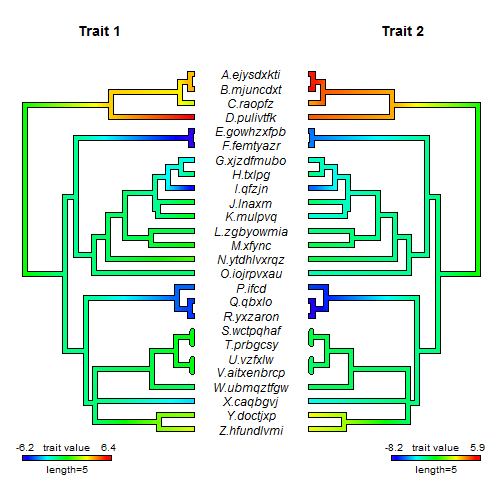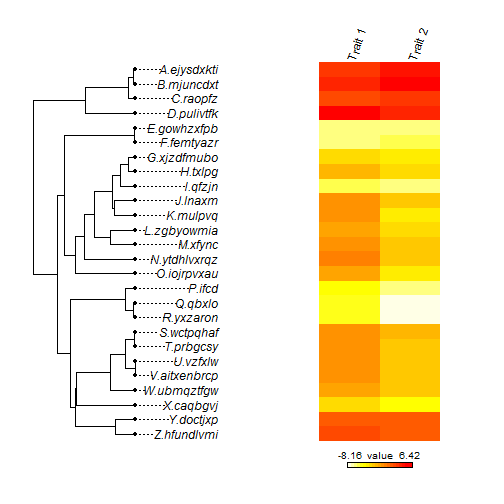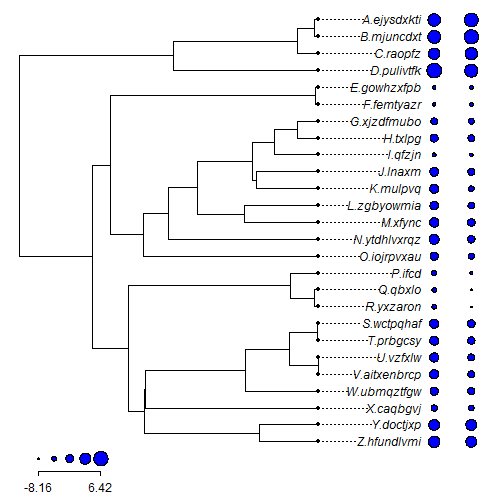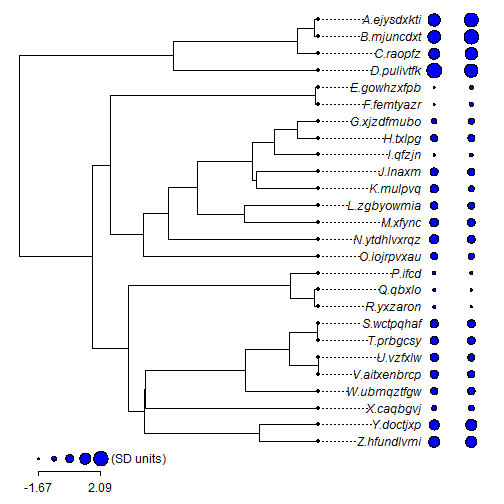## Wednesday, February 17, 2016

### Refresher on plotting facing `"contMap"` objects, and some updates to `plot` methods

I received a question recently about how to plot two facing `contMap` style trees. I'm pretty sure I've covered this before, but here's a quick refresher.

``````## load phytools
library(phytools)
packageVersion("phytools")
``````
``````##  '0.5.17'
``````
``````## here are my tree & data
tree
``````
``````##
## Phylogenetic tree with 26 tips and 25 internal nodes.
##
## Tip labels:
##  Z.hfundlvmi, Y.doctjxp, X.caqbgvj, W.ubmqztfgw, V.aitxenbrcp, U.vzfxlw, ...
##
## Rooted; includes branch lengths.
``````
``````X
``````
``````##                  Trait 1   Trait 2
## Z.hfundlvmi   2.92865937  2.184148
## Y.doctjxp     2.06631025  2.286198
## X.caqbgvj    -2.94735743 -3.818020
## W.ubmqztfgw  -0.73396217 -1.942123
## V.aitxenbrcp  0.26393810 -2.086957
## U.vzfxlw      0.25402396 -2.109128
## T.prbgcsy     0.16793804 -1.725199
## S.wctpqhaf    0.35749291 -1.392195
## R.yxzaron    -4.87172318 -8.161849
## Q.qbxlo      -4.90003166 -7.796955
## P.ifcd       -4.37436361 -6.676666
## O.iojrpvxau  -0.84578913 -3.085084
## N.ytdhlvxrqz  1.17336030 -1.695673
## M.xfync       0.52891770 -1.657742
## L.zgbyowmia  -0.41660675 -2.401744
## K.mulpvq      0.06040434 -3.719370
## J.lnaxm      -0.12707579 -2.148399
## I.qfzjn      -5.45744073 -6.189724
## H.txlpg      -1.06821601 -2.431826
## G.xjzdfmubo  -2.50951467 -3.482821
## F.femtyazr   -6.16411522 -5.756618
## E.gowhzxfpb  -6.04269227 -6.048308
## D.pulivtfk    6.42009980  4.654686
## C.raopfz      2.79829549  3.821099
## B.mjuncdxt    4.48219431  5.865553
## A.ejysdxkti   4.10171985  5.524872
``````
``````## create a layout for the trees & labels
layout(matrix(1:3,1,3),widths=c(0.4,0.2,0.4))
par(cex=1) ## make sure the correct font size is used in subplots
map1<-contMap(tree,X[,1],plot=FALSE) ## create "contMap" object
map1<-setMap(map1,invert=TRUE) ## invert color map
## plot "contMap" object
plot(map1,fsize=c(0,0.8),ftype=c("off","reg"),sig=1,legend=5,
xlim=c(-0.1,1)*max(nodeHeights(tree)),mar=c(1.1,0.1,4.1,0.1))
title(main=colnames(X))
## plot labels
ylim<-c(1-0.12*(length(tree\$tip.label)-1),length(tree\$tip.label))
plot.new()
plot.window(xlim=c(-0.1,0.1),ylim=ylim)
text(rep(0,length(tree\$tip.label)), 1:length(tree\$tip.label),tree\$tip.label,
font=3)
## repeat for trait 2
map2<-contMap(tree,X[,2],plot=FALSE)
map2<-setMap(map2,invert=TRUE)
plot(map2,fsize=c(0,0.8),ftype=c("off","reg"),
direction="leftwards", sig=1,legend=5,
xlim=c(-0.1,1)*max(nodeHeights(tree)),mar=c(1.1,0.1,4.1,0.1))
title(main=colnames(X))
``````1) I added user control of `xlim` in `plot.contMap` (and `plot.densityMap`). This is because I discovered that the legend was often being cut off for small plotting windows when `xlim` was set at its default values; and

2) I added the argument `"direction"` to `add.color.bar` which is used by `plot.densityMap` and `plot.contMap` internally to plot the legend. This has the effect of 'flipping' the direction of the color bar for left-facing trees which previously would have also been left-facing. (I don't think I noticed before.)

These data were simulated, obviously. The following gives the code I used for simulation:

``````## simulate a tree & make realistic looking tip labels
tip.label<-sapply(LETTERS[26:1],function(x) paste(x,".",
paste(sample(letters,round(runif(n=1,min=4,max=10))),collapse=""),
sep=""))
tree<-pbtree(n=26,tip.label=tip.label,scale=10)
## simulate correlated trait data
V<-matrix(c(1,0.8,0.8,1),2,2)
X<-sim.corrs(tree,V)
colnames(X)<-paste("Trait",1:2)
``````

Note that there are other functions now available to visualize multiple continuous characters on a tree, such as `phylo.heatmap`:

``````phylo.heatmap(tree,X,fsize=c(1,1,0.8))
``````(although it doesn't look great for just two traits), or `dotTree` (which does):

``````dotTree(tree,X)
````````````## or
dotTree(tree,X,standardize=TRUE)
``````1.I try to plot conMap tree with the equal branch length, but it always shows as phylogram with particular lenght of each branch.

Could you give me some suggestions to solve this problem?

2.This comment has been removed by the author.

3.Hello Liam,

Thanks a lot for this post, I'm using the contMap function to generate a two trees like in your first image. I have the problem that species are showed in alphabetical order and not according to their phylogenetic position.

Do you have any suggestions to resolve this problem?

4.